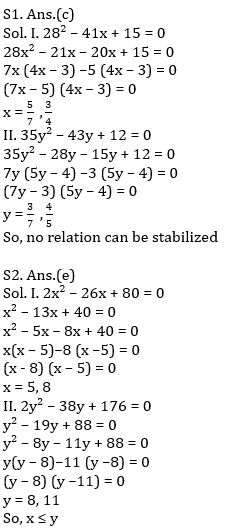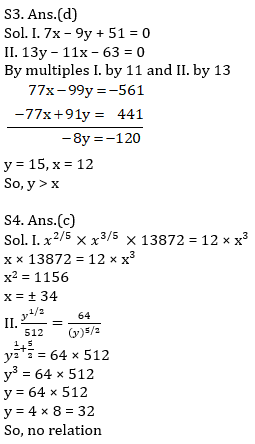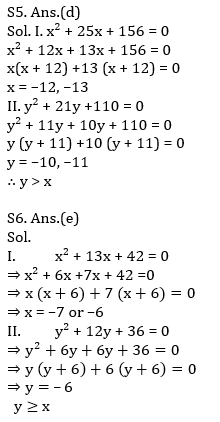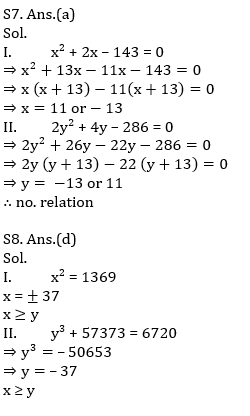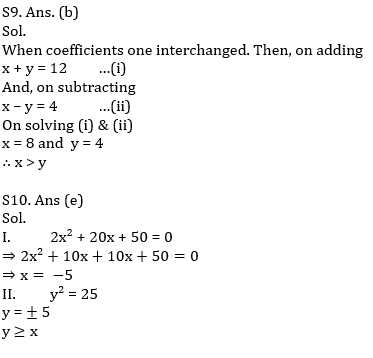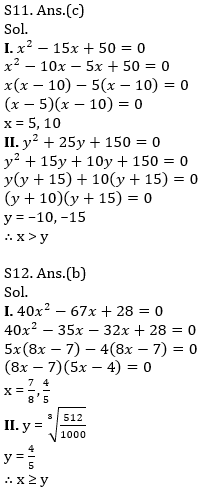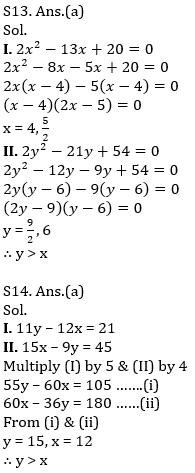Latest Banking jobs   »   Quantitative Aptitude Quiz For IBPS RRB...

# Quantitative Aptitude Quiz For IBPS RRB PO, Clerk Prelims 2021- 28th July

Directions (1–15): In each of these questions, two equations (I) and (II) are given. You have to solve both the equations and answer the following questions.
(a) x>y
(b) x≥y
(c) x=y or no relation.
(d) x<y
(e) x≤y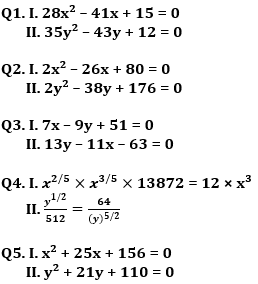Directions (6-10): In each question two equations numbered (I) and (II) are given. Student should solve both the equations and mark appropriate answer.
(a) If x=y or no relation can be established
(b) If x>y
(c) If x<y
(d) If x≥y
(e) If x≤y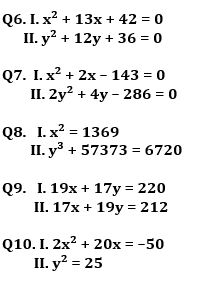Directions (11–15): In each of these questions, two equations (I) and (II) are given. You have to solve both the equations and answer the following questions.
(a) x < y
(b) x ≥ y
(c) x > y
(d) x ≤ y
(e) x = y or no relation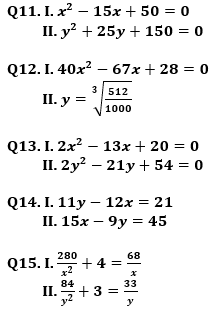Solutions